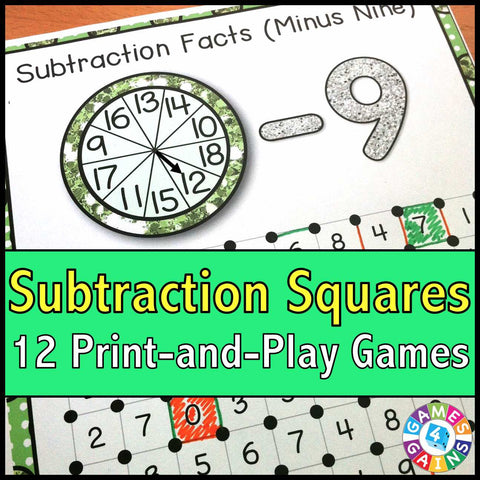Subtraction 'Squares' Game

• \$400

Subtraction 'Squares' Game contains 12 fun and engaging games to help students practice their subtraction facts.

Each one-page subtraction game gets students practicing a different set of subtraction facts (-1, -2, -3, etc). This means that you can have each student practicing the set of facts that he/she needs the most help with.

These "Subtraction Squares" games are so simple to use, and take a minimal amount of prep. Simply print out the game sheet, get a paperclip and fastener, and grab a few markers, and you'll be ready to go!

To play, students will spin the spinner and solve the subtraction problem. Then, they have to find the solution on the game board and draw a line between two of the dots surrounding that number. When a student's line forms a complete square, he/she gets to capture that square. The student with the most squares at the end of the game is the winner!

Included with this Subtraction 'Squares' Game:
Instructions for play
12 "Subtraction Squares" game boards (-0, -1, -2, -3, -4, -5, -6, -7, -8, -9, -10, mixed facts)